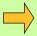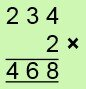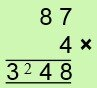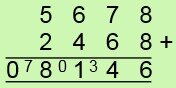Ten-year old Truman Henry Safford was asked:
"multiply in your head 365,365,365,365,365,365 by 365,365,365,365,365,365. He flew around the room like a top, pulled his pantaloons over the top of his boots, bit his hand, rolled his eyes in their sockets, sometimes smiling and talking, and then seeming to be in an agony, until, in no more than one minute, said he,
133, 491, 850, 208, 566, 925, 016, 658, 299, 941, 583, 225!"

Truman Henry Safford was born in America in 1836. He was a precocious child who took an interest in all subjects but especially mathematics and astronomy.
He became a professor of astronomy in 1876.

Chapter 1

On The Flag

Our mind has the capability to mark a figure, number or thought so that it stands out. We use this facility very frequently. In mental calculation we would like to hold the first part of the answer in mind and build it up step by step, from left to right, until it is complete. The technique introduced in this chapter for mental multiplication and addition is used in later chapters and is developed into the general multiplication method in chapter six.

Calculating from Left to Right

Since the figures in a number are normally spoken, written and read from left to right it is easier to calculate from left to right also. And since the usual methods of multiplication, addition and subtraction work from right to left it will be necessary for us to learn the alternative strategy of calculating from left to right. Once this is mastered however we will find that calculating from the left is easier and more natural. In the Vedic system all arithmetical operations can be carried out from left to right and this has important consequences which are briefly described at the end of this chapter and chapter six.Example 1

Suppose we want to multiply 234 by 2:Example 2

Now if we had 237 × 2:The products we get when we multiply 2,3,7 each by 2 (from left to right) are 4,6,14, and here the 6 and the 1 are merged together, to give 474 as the answer.

The 3 mental steps carried out, corresponding to multiplying each of the 3 figures by 2 are:
step 1:    4
step 2:    4,6 = 46
step 3:    4614 = 474.

The first part of the answer is held in the mind (On the Flag) and is built up digit by digit until the answer is complete.

In summary: if a single figure is to be combined with what is in memory (as in step 2 above) it is simply tagged on the end; if a double figure is to be combined (as in step 3 above) the left-hand digit is added to the number in memory and the right-hand digit is then tagged on the end. It is really very simple.Example 3

For 236 × 7:The mental steps are: 14
14,21 = 161
161,42 = 1652Example 4

4321 × 6 = 25926

The mental steps are:

24
24,18 = 258
258,12 = 2592
2592,6 = 25926Example 5

In multiplying 56 by 7: we get 35 (5×7), then 42 (6×7).

The 4 here is then added to the 35 to give 39 and the 2 is tagged on the end to give 392 as the answer.

You will find this simple technique easy and efficient, but you will need to practice it. The following exercise will help to establish the method.

After some practice with the sum in front of you you may like to try solving it after only a brief look at the sum.

Question Comment !
 Tutorial Title Question Title No Questions 0 Questions Asked 0 Skipped Questions 0 Correctly Answered 0 Wrongly Answered 0 Total Question Attempts 0 Current Question Attempts 0

Skip Question

Reset Test

Halt Test

 No Questions 0 Questions Asked 0 Skipped Questions 0 Correctly Answered 0 Wrongly Answered 0 Total Question Attempts 0

Time Taken

CloseExercise A

With practice you will find that the sums get easier. You will also find that your memory is strengthened and your mental agility is increased. But do not strain your mind, this will produce an adverse effect; the least force used in solving the sums the better.

Addition from Left to Right

This is just the same as multiplying from left to right.Example 6

Add 187 and 444.

We add up in each column. from left to right, and merge the totals together:The mental steps are: 5
5,12 = 62
62,11 = 631Example 7

If we have to add the numbers 45 and 78, we may think of the numbers one below the other. The total in the first column is 11, and the second column totals 13. The 1 in the 13 is then combined with the 11 to give 12 and the 3 is tagged on to this: 123. (11,13 = 123)

Addition of numbers is in frequent demand and this method will be found easy and fast once we have formed the habit of calculating from left to right through practice.Exercise B

This can of course be extended to the addition of longer numbers or to adding three or more numbers together, but since we are mostly unaccustomed to holding many figures in the head at once we will not take this any further here.

Subtraction from left to right and another addition device will be found in Chapter 5.

Writing Left to Right Calculations

For those interested in calculations done on paper, rather than mentally, the following procedure is probably the best.Example 8First we multiply the 8 by 4 to get 32.
The 3 is written in the answer and the 2 is put On the Flag in the next column.
Then 7×4 = 28, and the flagged 2 is combined with this 28 to give 48 (i.e. the flagged 2 counts as 20) and 48 is put down to complete the answer.Example 9Starting on the left 8×3 = 24: we put the 2 down as the first figure of the answer, and put the 4 On the Flag in the next column.
Then 7×3 = 21: 21 + flagged 4 (as 40) = 61. Put down 6, flag 1.
6×3 = 18: 18 + flagged 1 (as 10) = 28. Put down 2, flag 8.
1×3 = 3: 3 + flagged 8 (as 80) = 83. Put down 8, flag 3.
4×3 = 12: 12 + flagged 3 (as 30) = 42. Put down 42.

Alternatively the flagged numbers can be committed to memory rather than written down.Example 10In this addition sum we first get 5 + 2 = 7 and put down 0 in the answer and flag the 7. (The 0 can of course be left out).
6+4=10: 10+flagged 7=80. Put 8, flag 0.
7+6=13: 13+ flagged 0=13. Put 1, flag 3.
8+8=16: 16+ flagged 3=46. Put down 46.Exercise C

It has already been pointed out that calculating from left to right is easier and more natural as we write and pronounce numbers from the left. This makes mental calculations much simpler. It also means that we obtain the most significant figures in a calculation first: if we are multiplying a 6-figure number and we only want the first 3 figures of the answer it is a waste of time and effort to calculate the whole sum, which we would have to do in calculating the usual way from right to left. Other applications of this and an outline of more advanced applications in trigonometry etc. will be found in Chapter 6.